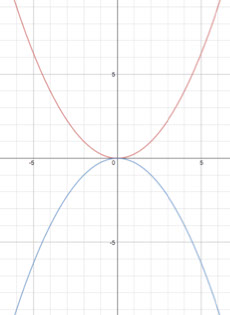# Parabolas Lesson 5 Episode 1 (Teachers)

### Making Sense

Sasha and Keoni examine the similarities and differences between two parabolas with vertices on the origin but with different distances to the focus. They determine if the same equation could represent both parabolas.

### Episode SupportsStudents’ Conceptual Challenges

Keoni wonders if a single equation (y = x2/4) could represent two different parabolas [1:34].

➤ Sasha disagrees with Keoni. They resolve their dispute by testing the coordinates of points on each of the parabolas in the given equation. They conclude that the two parabolas will have unique equations.

Focus Questions

For use in a classroom, pause the video and ask these questions:

1. [Pause video at 3:38]. What else do you notice about the given points and their relationships to the focus and the directrix of each parabola?

2. [Pause video at 5:04]. Which equation did Sasha just use? What does she mean by “it works”?

Supporting Dialogue

Focus students’ attention on conjecturing and providing evidence for their conjectures:

1. Name some other coordinates on the blue parabola. Explain how you know that they are on the blue parabola.

2. How might you predict other values on the blue parabola? What evidence are you using to make your predictions?

Math Extensions

1. Consider the two parabolas graphed below. How are they different? How are they the same?

2. Can you determine the focus and directrix of each parabola? Explain your thinking.